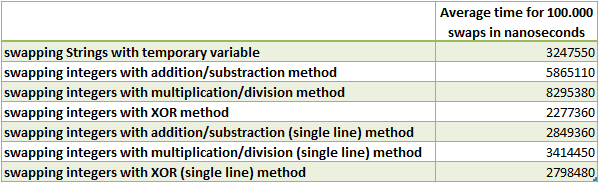## 1.概述

Sometimes, we may want to swap two variables in our code.

In this tutorial, we’ll see several ways to do that, depending on the types of variables we want to swap. Then, we will check the performances of each method.

## 2.简单的方法。使用一个临时变量

The simplest way to swap two variables is to use a third variable as temporary storage:

``````Object a, b;
Object temp;
temp = a;
a = b;
b = temp;``````

This method is particularly easy to read and understand, even for beginners. Its primary disadvantage is that it requires a temporary variable.

We should keep in mind that this method is the only one that can swap Object variables.

### 2.1.为什么不在一个方法中进行交换？

If we have to swap variables at several points in our code, it may sound attractive to create a method to swap variables like that:

``public void swap(Object a, Object b)``

Unfortunately, this won’t work in Java as references to objects are copied during method invocation.

If we really want to have a swap method, we have to use a wrapper class around your object and swap the object contained in the wrapper:

``````private class Wrapper {
public String string;
}``````

And the swap method:

``````private static void swap(Wrapper a, Wrapper b) {
String temp = b.string;
b.string = a.string;
a.string = temp;
}``````

With this method, enclosed Strings will remain swapped after the method returns.

## 3.不含临时变量

If our variables are of primitive types, we can find ways to swap them without temporary variables.

Let’s see several examples.

### 3.1.使用算术运算

We can use math to swap variables without temporary variables in several ways. For the following examples, let’s assume that we want to swap two integers a=5 and b=10.

We can use additions and subtraction for swapping:

``````a = a + b;  // a = 15
b = a - b;  // b = 5
a = a - b;  // a = 10``````

Or, we can use multiplications and divisions:

``````a = a * b;  // a = 50
b = a / b;  // b = 5
a = a / b;  // a = 10``````

We should keep in mind that this method doesn’t work if any of the numbers is 0 as the first operation will lead to storing a zero, making the rest of the algorithm useless. Moreover, if b=0, it will throw an ArithmeticException due to a division by zero.

We should also take care of primitives capacity as addition/multiplication can lead to numbers exceeding the maximum value of the primitive type. This may lead to errors after swapping without throwing any exception.

For example, if a = Integer.MAX_VALUE, then before swapping a=2147483647 and b=10 and after swapping, a=10, b=-1.

If we’re working with char, byte, or short types of data, an explicit cast is required as arithmetic operators result is a value of type int at least in Java:

``````a = (char)(a + b);
b = (char)(a - b);
a = (char)(a - b);``````

### 3.2.使用逻辑操作

If we’re working with integer data types (i.e., char, short, byte, int, long), we can use the exclusive OR bitwise operator (XOR). The “^” operator will process a bitwise XOR operation on all bits of our variables:

``````a = a ^ b;  // a = 1111 (15)
b = a ^ b;  // b = 1010 (5)
a = a ^ b;  // a = 0101 (10)
``````

We should be aware that, as for the arithmetic operators, the bitwise XOR operator returns at least int data type. So, we have to cast the result of the XOR for each line if we’re working with chars, bytes, or shorts variables.

### 3.3.单线变体

We can use a single-line version of the swapping methods to reduce the code size:

``````b = (a + b) – (a = b);
a += b – (b = a);
a = a * b / (b = a);
a = a ^ b ^ (b = a);``````

This works because expressions are evaluated with respect of the precedence of the operators. If a = 5 and b = 10 initially, the last expression is equivalent to a = 5 ^ 10 ^ (b = 5). The first operation (5 ^ 10) is exactly the first line of the multi-line algorithm, then we assign 5 to b (parenthesis have priority), and finally, we calculate 15 ^ 5 which is exactly the third line of the algorithm.

## 4.性能分析

We just saw that there are several ways to swap two variables in Java, but which one is the more efficient? To give a tendency on performances of each algorithm, we performed loops of variable swapping methods and measured the time needed to swap two variables 100.000 times. We ran the test 10 times to calculate the average execution time of each algorithm. Here are the results:The absolute time is not important here as it depends on the machine which is running the test. We only see that some algorithms are slower than others. It’s especially true for the multiplication/division one, which is significantly slower, either in its single-line version. On the opposite, the XOR algorithm is the most efficient in both multi and single-line versions.

Swapping Objects with a temporary variable is also quite efficient, which is quite understandable as only pointers are manipulated in that case.

## 5.总结

In this article, we looked at how to swap two variables in Java, depending on the type of the variables.

We described how to swap Objects, and then we studied several ways to swap primitives types with several algorithms. Finally, we had a look at the performances of each method.

As always, the source code for all examples is available over on GitHub.